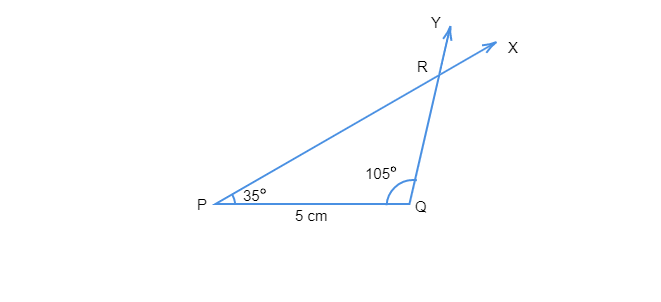# Construct $Ă˘Ë†â€ PQR$ if $PQ = 5\ cm$, $m\angle\ PQR = 105^{\circ}$ and $m\angle QRP = 40^{\circ}$. [Hint: Recall angle-sum property of a triangle.]

Given: $PQ = 5\ cm$, $m\angle\ PQR = 105^{\circ}$ and $m\angle QRP = 40^{\circ}$.

To do:  To construct $\triangle PQR$.

Solution:

Here given, $PQ = 5\ cm$, $m\angle PQR = 105^{\circ}$ and $m\angle QRP = 40^{\circ}$

Let us find the value of $\angle QPR=?$

$\angle PQR + \angle QRP + \angle QPR = 180^{\circ}$   [angle sum property of a triangle]

$\Rightarrow 105^{\circ} + 40^{\circ} + \angle QPR = 180^{\circ}$

$\Rightarrow \angle QPR = 35^{\circ}$

Steps of construction :

• Let us draw a line segment $PQ=5\ cm$.
• At $P$, let us draw a ray $PX$ making an angle of $35^{\circ}$ such that $\angle QPX=35^{\circ}$.
• At $Q$, let us draw a ray $QY$ making an angle of $105^{\circ}$ such that $\angle PQR=105^{\circ}$.
• Here, rays $PX$ and $QY$ intersect each other at the point $R$.$\triangle PQR$ is the required triangle.